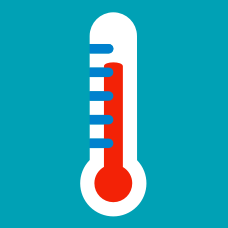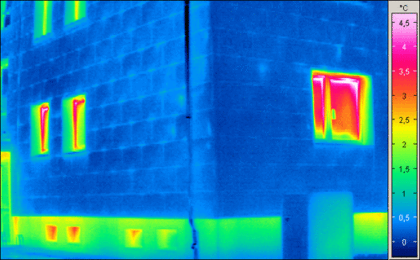Classical Mechanics

Zeroth law of thermodynamicsInitially, there are two very small, thermally isolated rooms $A$ and $B.$ The heat capacitance of room $A$ is $40$ quanta of energy and room $A$ contains $32$ quanta of energy. The heat capacitance of room $B$ is $100$ quanta of energy and room $B$ contains $50$ quanta of energy. When the two room are thermally connected, in which direction will the quanta flow, on average?

Calculate the temperature increase when $400 \text{ J}$ of heat is applied to $46.8 \text{ g}$ of $\ce{NaCl}.$

 Details and assumptions:

• The molar heat capacity for $\ce{NaCl}$ is $C_p = 50 \text{ J} \cdot \text{mol}^{-1} \cdot \text{K}^{-1} .$
• The formula weight of $\ce{NaCl}$ is $58.5 \text{ g/mol}.$

Initially, there are two thermally isolated rooms $A$ and $B.$ The heat capacitance of room $A$ is $80$ quanta of energy and room $A$ contains $56$ quanta of energy. The heat capacitance of room $B$ is $80$ quanta of energy and room $B$ contains $24$ quanta of energy.

Question: When the two rooms are thermally connected and allowed to approach equilibrium, how many quanta of energy are transferred, on average?

There are 2 beakers of water in a room. In one beaker, the temperature of water is $11 ^\circ \text{C}$ higher than room temperature, and the other is $11 ^\circ \text{C}$ lower than room temperature. Let them be on a table without contact with each other, then after enough time passes an equilibrium is reached. What is the difference between the temperatures of the waters in the beakers?

Two objects $A$ and $B$ with temperatures $T_1 = 258 \text{ K}$ and $T_2 = 269 \text{ K},$ respectively, are each in a closed system. They are brought into thermal contact and enough time passes. If the final temperature of both objects is $T_f,$ what is the relation between $T_1,$ $T_2$ and $T_f?$

×